# NCERT Solutions for Class 11 Chemistry Structure of Atom Part 2## myCBSEguide App

CBSE, NCERT, JEE Main, NEET-UG, NDA, Exam Papers, Question Bank, NCERT Solutions, Exemplars, Revision Notes, Free Videos, MCQ Tests & more.

NCERT solutions for Class 11 Chemistry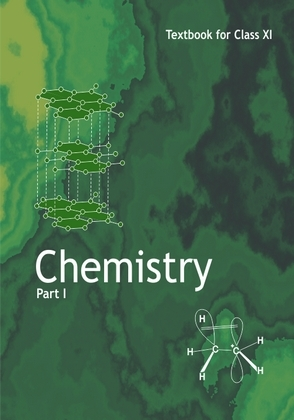## NCERT Class 11 Chemistry Chapter wise Solutions

• Chapter 1 – Some Basic Concepts of Chemistry
• Chapter 2 – Structure of Atom
• Chapter 3 – Classification of Elements and Periodicity in Properties
• Chapter 4 – Chemical Bonding and Molecular Structure
• Chapter 5 – States of Matter
• Chapter 6 – Thermodynamics
• Chapter 7 – Equilibrium
• Chapter 8 – Redox Reactions
• Chapter 9 – Hydrogen
• Chapter 10 – The s-Block Elements
• Chapter 11 – The p-Block Elements
• Chapter 12 – Organic Chemistry – Some Basic Principles and Techniques
• Chapter 13 – Hydrocarbons
• Chapter 14 – Environmental Chemistry

## NCERT Solutions for Class 11 Chemistry Structure of Atom Part 2

Class 11 Chemistry Structure of Atom Part 2

17. Calculate the wave number for the longest wavelength transition in the Balmer series of atomic hydrogen

18. What is the energy in joules, required to shift the electron of the hydrogen atom from the first Bohr orbit to the fifth Bohr orbit and what is the wavelength of the light emitted when the electron returns to the ground state? The ground state electron energy is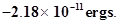19. The electron energy in hydrogen atom is given by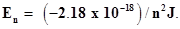Calculate the energy required to remove an electron completely from then= 2 orbit. What is the longest wavelength of light in cm that can be used to cause this transition?

20. Calculate the wavelength of an electron moving with a velocity of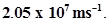21. The mass of an electron is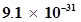kg. If its K.E. is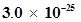J, calculate its wavelength.

22. Which of the following are isoelectronic species i.e., those having the same number of electrons?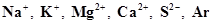23. (i) Write the electronic configurations of the following ions: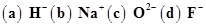(ii) What are the atomic numbers of elements whose outermost electrons are represented by (a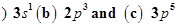?

(iii) Which atoms are indicated by the following configurations?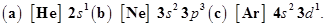24. What is the lowest value of n that allows g orbitals to exist?

25. An electron is in one of the 3d orbitals. Give the possible values of n, l and ml for this electron.

26. An atom of an element contains 29 electrons and 35 neutrons. Deduce (i) the number of protons and (ii) the electronic configuration of the element.

27. Give the number of electrons in the species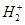,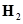and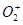28. (i) An atomic orbital has n = 3. What are the possible values of l and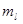?

(ii) List the quantum numbers (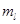and l) of electrons for 3d orbital.

(iii) Which of the following orbitals are possible?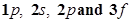29. Using s, p, d notations, describe the orbital with the following quantum numbers.

(a) n = 1, l = 0;

(b) n = 3; l =1

(c) n = 4; l = 2;

(d) n = 4; l=3.

30. Explain, giving reasons, which of the following sets of quantum numbers are not possible.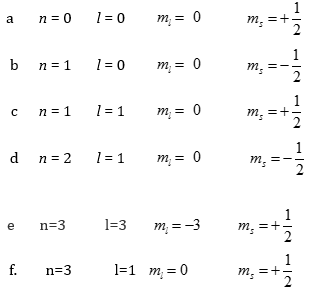31. How many electrons in an atom may have the following quantum numbers?

(a)n = 4,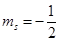(b) n = 3, l = 0

32. Show that the circumference of the Bohr orbit for the hydrogen atom is an integral multiple of the de Broglie wavelength associated with the electron revolving around the orbit.

## NCERT Solutions for Class 11 Chemistry Chapter 2 Structure of Atom Part 2

NCERT Solutions Class 11 Chemistry Structure of Atom Part 2 PDF (Download) Free from myCBSEguide app and myCBSEguide website. Ncert solution class 11 Chemistry includes text book solutions from both part 1 and part 2. NCERT Solutions for CBSE Class 11 Chemistry have total 14 chapters. 11 Chemistry NCERT Solutions in PDF for free Download on our website. Ncert Chemistry class 11 solutions PDF and Chemistry ncert class 11 PDF solutions with latest modifications and as per the latest CBSE syllabus are only available in myCBSEguide

## NCERT Solutions for Science Class 3rd to 12th

To download NCERT Solutions for class 11 Chemistry, Physics, Biology, History, Political Science, Economics, Geography, Computer Science, Home Science, Accountancy, Business Studies and Home Science; do check myCBSEguide app or website. myCBSEguide provides sample papers with solution, test papers for chapter-wise practice, NCERT solutions, NCERT Exemplar solutions, quick revision notes for ready reference, CBSE guess papers and CBSE important question papers. Sample Paper all are made available through the best app for CBSE students and myCBSEguide.com website.